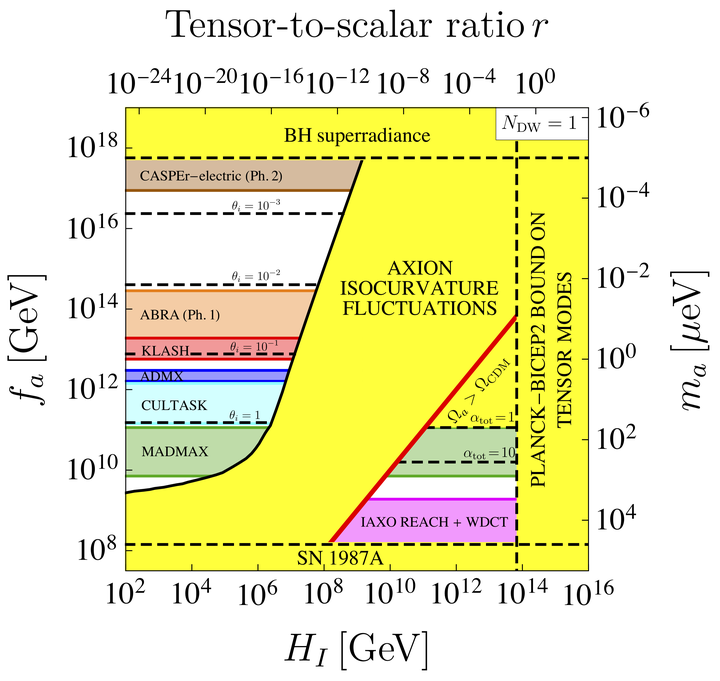# Axion cold dark matter in view of BICEP2 results### Abstract

The properties of axions that constitute 100% of cold dark matter (CDM) depend on the tensor-to-scalar ratio $r$ at the end of inflation. If $r=0.20^{+0.07}_{-0.05}$ as reported by the BICEP2 collaboration, then half’’ of the CDM axion parameter space is ruled out. Namely, the Peccei-Quinn symmetry must be broken after the end of inflation, and axions do not generate non-adiabatic primordial fluctuations. The cosmic axion density is then independent of the tensor-to-scalar ratio $r$, and the axion mass is expected to be in a narrow range that however depends on the cosmological model before primordial nucleosynthesis. In the standard $\Lambda$CDM cosmology, the CDM axion mass range is $m_a = \left(71 \pm 2\right) \mu{\rm eV} , (\alpha^{\rm dec}+1)^{6/7}$, where $\alpha^{\rm dec}$ is the fractional contribution to the cosmic axion density from decays of axionic strings and walls.

Publication
Physical Review Letters 113, 011802 (2014)
Click the Cite button above to demo the feature to enable visitors to import publication metadata into their reference management software.
Click the Slides button above to demo Academic’s Markdown slides feature.

Supplementary notes can be added here, including code and math.##### Luca Visinelli
###### Tenure-track Associate Professor, Shanghai Jiao Tong University; Fellow, Tsung-Dao Lee Institute

PhD in Physics, working on Particle Astrophysics and Dark Matter modeling.